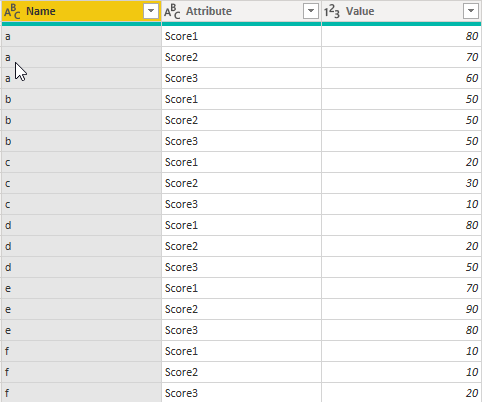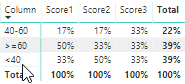cancel
Showing results for
Did you mean:

Fabric is Generally Available. Browse Fabric Presentations. Work towards your Fabric certification with the Cloud Skills Challenge.Helper II

## Creating a matrix with multiple measures to display a distribution

Hi all,

I have trouble creating a matrix where I would like to put different measures I calculated to show a distribution.

I have this first table where I can see the names of the people and their score to 3 different tests:

 Name Score1 Score2 Score3 a 80 70 60 b 50 50 50 c 20 30 10 d 80 20 50 e 70 90 80 f 10 10 20

I would like to create a matrix where I can see the distribution of each score under it, that would look like this :

 Score1 Score2 Score3 >=60 50% 33% 33% 40-60 17% 17% 33% <40 33% 50% 33%

For now, I can calculate each of the value, but I don't know how to integrate them in one table.

The measures I use are this, for each score:

Score1 60 and up = CALCULATE(COUNT('Sheet1'[userid]);FILTER('Sheet1';'Sheet1'[Score1]>=60)

Score1 40-60 = CALCULATE(COUNT('Sheet1'[userid]);FILTER('Sheet1';'Sheet1'[Score1]>=40);'Sheet1'[Score1]<60)
Score1 40 and below = CALCULATE(COUNT('Sheet1'[userid]);FILTER('Sheet1';'Sheet1'[Score1]<40)

Does someone know how to do this?

1 ACCEPTED SOLUTIONSolution Sage

Hi @MylèneB ,

In your scenario, we need to unpivot the table using the following query in Edit Queries:

```let
Source = Table.FromRows(Json.Document(Binary.Decompress(Binary.FromText("i45WSlTSUbIwABLmIMLMQClWJ1opCcg0NUAiQILJQKYRiG8MIgwhgikw7UZIKlNhxlkaQKRBgmkQTVACqDw2FgA=", BinaryEncoding.Base64), Compression.Deflate)), let _t = ((type text) meta [Serialized.Text = true]) in type table [Name = _t, Score1 = _t, Score2 = _t, Score3 = _t]),
#"Changed Type" = Table.TransformColumnTypes(Source,{{"Name", type text}, {"Score1", Int64.Type}, {"Score2", Int64.Type}, {"Score3", Int64.Type}}),
#"Unpivoted Other Columns" = Table.UnpivotOtherColumns(#"Changed Type", {"Name"}, "Attribute", "Value")
in
#"Unpivoted Other Columns"```

Then we will get a table like below:Then we can create a calculated column using the following DAX query:

```Column =
IF (
'Table'[Value] >= 60,
">=60",
IF ( 'Table'[Value] >= 40 && 'Table'[Value] < 60, "40-60", "<40" )
)```

After that, we can create a measure using the following DAX query:

```Measure 2 =
DIVIDE (
COUNTROWS ( 'Table' ),
CALCULATE ( COUNTROWS ( 'Table' ), ALL ( 'Table'[Column] ) )
)```

Then drag the fields and measure to matrix, we will get a table like below:Here is the pbix file, please refer to it: https://1drv.ms/u/s!AjytBuzIG5m9ti-8yXcTvKacfZLN

Best Regards,

Teige

2 REPLIES 2Solution Sage

Hi @MylèneB ,

In your scenario, we need to unpivot the table using the following query in Edit Queries:

```let
Source = Table.FromRows(Json.Document(Binary.Decompress(Binary.FromText("i45WSlTSUbIwABLmIMLMQClWJ1opCcg0NUAiQILJQKYRiG8MIgwhgikw7UZIKlNhxlkaQKRBgmkQTVACqDw2FgA=", BinaryEncoding.Base64), Compression.Deflate)), let _t = ((type text) meta [Serialized.Text = true]) in type table [Name = _t, Score1 = _t, Score2 = _t, Score3 = _t]),
#"Changed Type" = Table.TransformColumnTypes(Source,{{"Name", type text}, {"Score1", Int64.Type}, {"Score2", Int64.Type}, {"Score3", Int64.Type}}),
#"Unpivoted Other Columns" = Table.UnpivotOtherColumns(#"Changed Type", {"Name"}, "Attribute", "Value")
in
#"Unpivoted Other Columns"```

Then we will get a table like below:Then we can create a calculated column using the following DAX query:

```Column =
IF (
'Table'[Value] >= 60,
">=60",
IF ( 'Table'[Value] >= 40 && 'Table'[Value] < 60, "40-60", "<40" )
)```

After that, we can create a measure using the following DAX query:

```Measure 2 =
DIVIDE (
COUNTROWS ( 'Table' ),
CALCULATE ( COUNTROWS ( 'Table' ), ALL ( 'Table'[Column] ) )
)```

Then drag the fields and measure to matrix, we will get a table like below:Here is the pbix file, please refer to it: https://1drv.ms/u/s!AjytBuzIG5m9ti-8yXcTvKacfZLN

Best Regards,

TeigeHelper II

Thank you so much, it worked really well!

As a second step I would want to be able to filter this matrix with slicers.

For example, I have different persons in different groups and I would like to be able to look only at group A and that my distribution matrix is also measuring only group A, is it possible to do this?Announcements#### Power BI Monthly Update - November 2023

Check out the November 2023 Power BI update to learn about new features.#### Fabric Community News unified experience

Read the latest Fabric Community announcements, including updates on Power BI, Synapse, Data Factory and Data Activator.#### Exclusive opportunity for Women!

Join us for a free, hands-on Microsoft workshop led by women trainers for women where you will learn how to build a Dashboard in a Day!#### The largest Power BI and Fabric virtual conference

130+ sessions, 130+ speakers, Product managers, MVPs, and experts. All about Power BI and Fabric. Attend online or watch the recordings.Top Solution Authors
Top Kudoed Authors
Users online (4,859)